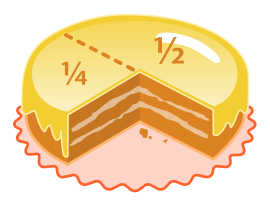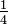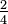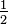# Fraction (mathematics) facts for kids

Kids Encyclopedia FactsA cake with one quarter removed. The remaining 34 of the cake has been divided into two pieces.

A fraction is a number that shows how many equal parts there are. When we write fractions, we show one number with a line above another number, for example,$\tfrac{1}{4}$ or 14 or 1/4. The top number tells us how many parts there are, the second number tells us the total number of parts.

The top part of the fraction is called a numerator. The bottom part of the fraction is called a denominator. For example,$\tfrac{1}{4}$: The 1 is the numerator here, and the 4 is the denominator.

## Examples

1. For example, a room where$\tfrac{1}{4}$ of the people are girls, has 1 girl for every 4 people.
2. Think of a cake made of 4 equal parts. Each is 1 part of 4. In maths, you write this as$\tfrac{1}{4}$, and it is called a "quarter". 2 parts of the cake (i.e. 2 quarters) is written$\tfrac{2}{4}$, and this is also known as 1/2, half.

## Mathematical fractions

A fraction is a mathematical expression relating two quantities or numbers, one divided by the other. The numbers may be whole numbers (or integers) – this is a rational number. For example,$\tfrac{1}{2}$ is a fraction. They can also be polynomials – this is a rational function.

Mathematically, a fraction is a quotient of numbers, representing the number's value when the numerator is divided by the denominator (lower number). Thus$\tfrac{1}{2}$ means one divided by two, or, in decimals, 0.5.

To find$\tfrac{1}{2}$ of$\tfrac{1}{2}$, the denominators are multiplied. That means: denominator 2 multiplied by 2 equals 4 or:$\tfrac{1}{2}$ x$\tfrac{1}{2}$ =$\tfrac{1}{4}$ or: 0.5 x 0.5 = 0.25.

(In this case “$\tfrac{1}{2}$ of” means mathematically "multiplication".)

To find$\tfrac{1}{2}$ divided by$\tfrac{1}{2}$, multiply$\tfrac{1}{2}$ by the reciprocal of$\tfrac{1}{2}$, which is 2. That answer is 1.

## Multiplying

The numerator is multiplied by the other numerator. The denominator is multiplied by the other denominator. E.g. 24 x 34 = 616 you can simplify this by dividing both the numbers by a common factor.This would be 38Fraction (mathematics) Facts for Kids. Kiddle Encyclopedia.